Next: Nucleon-Nucleon Interaction Up: maub-42w Previous: Nucleon-Pion Lagrangian

# FEW-NUCLEON SYSTEMS

In the previous section we have obtained Lagrangian densities that describe composite particles like pions (38) or nucleons (44). These particles are built of the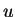and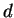valence quarks as well as of virtual gluons and quark-antiquark pairs. In fact, we can say that the virtual constituents provide for binding of the valence constituents, and most of the rest mass of composite particles comes from the binding by virtual constituents. Indeed, the rest masses of pions,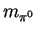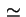135MeV and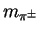140MeV, and nucleons,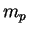938MeV and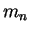940MeV, are much, much larger than those of quarks,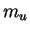3MeV and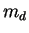6MeV. Moreover, the famous confinement effect prevents the valence quarks from being separated one from another, unless a real quark-antiquark pair is created from the vacuum, and two separate composite particles appear.

It is amazing that strong interactions only yield a strong binding for objects that cannot be broken apart at all. Once these composite particles are built, strong interactions become almost completely saturated, and what remains of them, when looked upon from the outside of composite particles, is in fact a relatively weak force.

Let us illustrate this weakness of the strong force by several examples. First of all, the only bound binary system of nucleons, i.e., the deuteron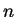-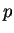, has the binding energy of only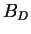=2.224575(9)MeV. This is really a small number as compared to, e.g., either the nucleon rest masses, or the QCD coupling constant. The deuteron is barely bound, and moreover, neither the di-neutron (-), nor the di-proton (-) is a bound object. The-scattering amplitude has a pole (corresponding to the deuteron bound state) at the relative momentum of only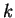=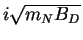45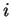MeV (the pole appears on the imaginary axis). The corresponding scattering length is fairly large,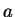=5.424(3)fm, and certainly much larger than the size of the deuteron,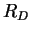=1.953(3)fm. The range of the nucleon-nucleon (NN) interaction, as given by the OPE potential, corresponds to the inverse of the pion mass 1/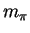, and equals to about 1.4fm [note that in the units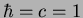we have 1fm(197MeV)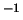].

The above scattering characteristics pertain to the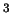S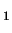channel, i.e., to a scattering with the total spin of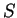=1, the total orbital angular momentum of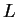=0, the total angular momentum of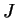=1, and the total isospin of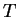=0. In the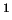S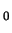channel (=0,=0,=0, and=1) the deuteron is unbound, the scattering amplitude has a pole at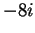MeV (on the negative imaginary axis - corresponding to the so-called virtual, or quasibound state), and the scattering length is very large negative,=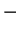23.749(8)fm. The-and-scattering lengths (in theSchannel) are also very large negative,=18.5(4)fm and=7.806(3)fm, respectively. Finally, the multi-nucleon bound objects (i.e., the atomic nuclei) are also very weakly bound, with the binding energy per nucleon of only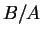8MeV.

These weak bindings have very important consequences for the physics of nuclear systems, namely, in these systems, the average kinetic energies are large positive, and the average potential (interaction) energies are large negative. The resulting total energies are therefore much smaller than either the kinetic or the potential component. As a result, one can neither treat the interaction as a small perturbation on top of the (almost) free motion of constituents, nor treat the relative kinetic energy as a small perturbation on top of a tightly bound, frozen system.

SubsectionsNext: Nucleon-Nucleon Interaction Up: maub-42w Previous: Nucleon-Pion Lagrangian
Jacek Dobaczewski 2003-01-27